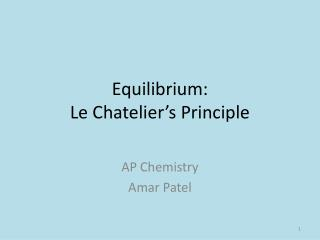# Equilibrium: Le Chatelier’s Principle - PowerPoint PPT PresentationDownload PresentationEquilibrium: Le Chatelier’s Principle

Equilibrium: Le Chatelier’s PrincipleDownload Presentation## Equilibrium: Le Chatelier’s Principle

- - - - - - - - - - - - - - - - - - - - - - - - - - - E N D - - - - - - - - - - - - - - - - - - - - - - - - - - -
##### Presentation Transcript

1. Equilibrium:Le Chatelier’s Principle AP Chemistry Amar Patel

2. Purpose To see the effect of changes of concentration on an equilibrium system

3. The equation of our equilibrium system is: Cu(H2O)52+(aq) + 4Cl-(aq) ⇌ CuCl42-(aq) +5H2O(aq) - This is the net ionic equation

4. Safety • Wear goggles at all times • Use stoppers when shaking test tubes • Do NOT directly smell or touch the chemicals • Do NOT ingest the chemicals • Be careful with glass test tubes

5. The equation of our equilibrium system is: Cu(H2O)52+(aq) + 4Cl-(aq) ⇌ CuCl42-(aq) +5H2O(aq) - This is the net ionic equation

6. Cu(H2O)52+(aq) + 4Cl-(aq) ⇌ CuCl42-(aq) +5H2O(aq) • Le Chatelier’s Principle: When a stress is placed on a system at equilibrium, the system will react (shift) in a direction to relieve the stress

7. Stress 1: Concentration Cu(H2O)52+(aq) + 4Cl-(aq) ⇌ CuCl42-(aq) +5H2O(aq) What is the Kc expression?

8. Cu(H2O)52+(aq) + 4Cl-(aq) ⇌ CuCl42-(aq) +5H2O(aq) What is the Kp? [CuCl42-][H2O]5 Kc= [Cu(H2O)52+][Cl-]4

9. Cu(H2O)52+(aq) + 4Cl-(aq) ⇌ CuCl42-(aq) + 5H2O(aq)Predict the equilibirum shift and effect on [CuCl42- ]. • Adding Cu(H2O)52+ • Adding 4Cl- • Adding H2O • Removing Cu(H2O)52+ • Removing CuCl42- • Adding Ne gas • Adding AgNO3 • Right; Increase • Right; Increase • Left; Decrease • Left; Decrease • Right; Increase** • No effect – Why? • Left; Decrease – Why?

10. Stress 2: Temperature New equilibrium system is: Co(H2O)62+(aq) + 6Cl-(aq) ⇌ CoCl62-(aq) + 6H2O(aq)

11. Is the reaction endothermic or exothermic? Co(H2O)62+(aq) + 6Cl-(aq) ⇌ CoCl62-(aq) + 6H2O(aq) We can treat heat/energy as a reactant or product. Think about the direction of the shift. Is heat a reactant or product?

12. Temperature • The shift will occur to correct the stress (removal or addition of heat). • Remember that K is temperature dependent so K will change with changes in temperature. • Endothermic rxn with heat input • Heat is a reactant. • By adding heat, the rxn shifts to the product side • K (products divided by reactants) increases

13. Stress 3: Pressure/Volume New equilibrium system is: PCl3(g) + Cl2(aq) ⇌ PCl5(g) Remember Boyle’s Law? What is the relationship between P and V? Inverse relation – As P increases, V decreases

14. Pressure/Volume • If there is a increase in pressure (decrease in volume), the system will try to reduce the pressure by shifting to decrease the number of gas molecules PCl3(g) + Cl2(aq) ⇌ PCl5(g) Left has 2 molecules, right has 1 molecule so the reaction will shift to the right • If both sides of rxn have same number of molecules, change in P has NO EFFECT.

15. Examples • CO (g) + H2O(g) ⇌ CO2(g) + H2 (g) a.) What is the Kc expression? b.) If [CO2 ] increases, then [H2O] _______ c.) If [H2] decreases, then [CO] ________

16. [CO2][H2] CO (g) + H2O(g) ⇌ CO2(g) + H2 (g) Kc= [CO][H2O] b.) If [CO2 ] increases, then [H2O] _______ c.) If [H2] decreases, then [CO] ________

17. Examples 2. Fe(s) + H2O(g) ⇌ Fe3O4 (S) + H2 (g) ∆H⁰ = -155 kJ a.) Balance the equation. b.) Write the Kcexpression for the equation. How will each of the following affect the concentration of the H2 (increase, decrease, or unchanged) in an equilibrium mixture of the reaction at 100⁰C : c.) Raise the temperature to 250⁰ d.) Remove the amount of Fe3O4 (S) e.) Add a small amount of H2O(g)

18. [H2]4 3 Fe(s) + 4 H2O(g) ⇌ Fe3O4 (S) + 4 H2 (g) ∆H⁰ = -155 kJ Kc= [H2O]4 How will each of the following affect the concentration of the H2 (increase, decrease, or unchanged) in an equilibrium mixture of the reaction at 100⁰C : c.) Raise the temperature to 250⁰ d.) Remove the amount of Fe3O4 (S)NO CHANGE e.) Add a small amount of H2O(g)